TOP > Contamination Control > The Mechanism of Particle adhesion

# The Mechanism of Particle adhesion

## Adhesion is caused by static electricity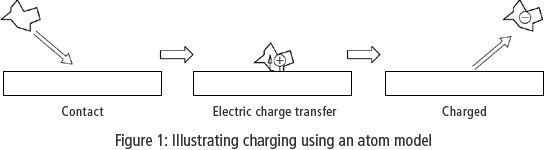When dust is carried on air currents generated by air conditioning and similar devices, the dust takes on a positive or negative static electric charge due to contact with various objects. Dust that has a positive electric charge will be attracted to objects that have a negative electric charge, and vice versa. The greater the amount of dust in the air, the larger the amount of dust that clings to objects within the room.
Also, if sources of dust (mainly people and clothing) are electrically charged, the dust that is generated from these sources is electrically charged as well. This attractive force generated by static electricity is known as “Coulomb force.” Coulomb force is expressed by the following formula: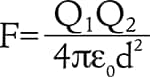Where F [N] is the Coulomb force, Q1 and Q2 [C] are electric charges, d [m] is the distance between the electric charges, and ε0 [F/m] is the electric constant (ε0 = 8.85 × 10-12 F/m) The Coulomb force is stronger when the amount of static electricity present in the objects is larger, and is weaker as the distance between the objects becomes greater. If the distance is multiplied by 2, the Coulomb force becomes 1/4 of its previous value. If the distance is multiplied by 10, the Coulomb force becomes 1/100 of its previous value. This means that the force that acts between two objects separated by 1 mm 0.04" is reduced to 1/100 of its previous strength when the objects are separated by 10 mm 0.39".

## Particle clinging to particle

We calculated the distance at which two spheres that are charged as much as possible begin to cling together due to the Coulomb force.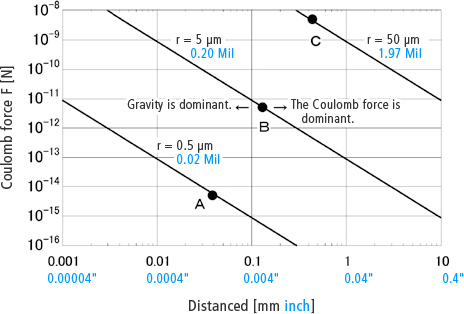Distance is displayed on the horizontal axis, and Coulomb force is displayed on the vertical axis. The three lines indicate three examples that each have different particle sizes. The three types of particles have diameters of 0.5 μm 0.02 Mil, 5 μm 0.20 Mil, and 50 μm 1.97 Mil. Let’s concentrate on points A, B and C. These points indicate the distances at which the strength of the Coulomb force acting between the particles and the strength of gravity acting on the particles are in equilibrium. This means that if the particles come any closer to each other than this distance, they will cling together due to the Coulomb force being stronger than gravity. If the distance is greater than that indicated by the points, gravity will supersede the Coulomb force, which will cause the particles to fall to the ground without clinging to each other. The 5 μm 0.20 Mil particles are attracted to each other at a distance of less than 0.1 mm 0.004". At a distance greater than or equal to 0.1 mm 0.004", the particles fall to the ground without clinging to each other.
As such, it can be understood that particles only cling to each other due to static electricity when they are separated by extremely short distances.

## Particle clinging to objects

The magnitude of the Coulomb force is also changed by the amount of static electricity. In other words, the strength of the Coulomb force changes depending on what object is charged with static electricity. Because particles have a small amount of static electricity, they only cling together at short range. Let us consider the case in which both a piece of dust and an object are charged with static electricity. The object is much larger than the piece of dust, so the object has a larger amount of static electricity. Also, if the object is large, the method for attenuating the Coulomb force is no longer inversely proportional to the square of the distance. We have omitted the detailed calculations, but if the object is charged with static electricity, it may attract dust from a distance of tens of centimeters.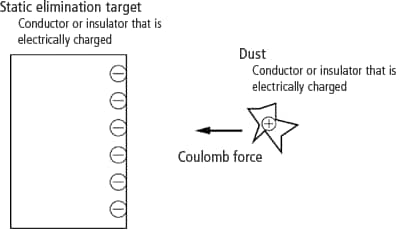Next, let us consider the case in which the object is not electrically charged but the piece of dust is. In this case, it is necessary to consider one more condition: the conductivity of the object. First, consider a conductor. When the electrically charged piece of dust approaches the conductor, an electric charge with the opposite polarity of the static electricity of the piece of dust is drawn out from the side of the conductor close to the piece of dust. This is caused by the movement of electric charges within the conductor. This phenomenon is called electrostatic induction.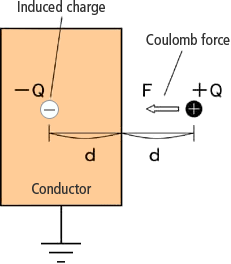Grounding the object does not work as a countermeasure for this phenomenon. Doing so does not prevent the phenomenon of the opposite electric charge being drawn out due to the Coulomb force. The only countermeasure is the elimination of static electricity from the dust. The surface of a conductor and dust have electric charges with opposite polarities, so the Coulomb force is generated.
As before, we calculated the distance at which a piece of dust is attracted to an object.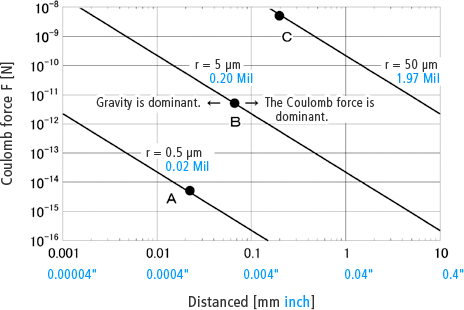For a particle with a diameter of 5 μm 0.20 Mil, the Coulomb force becomes strong and the particle clings to the object at a distance of 0.1 mm 0.004" or less. Giving some leeway, the particle does not cling to the object if they are separated by at least a few millimeters.
However, only the piece of dust is electrically charged and the electric charge is small, so the Coulomb force is weak and the distance at which the dust clings to the object is short, just a few millimeters.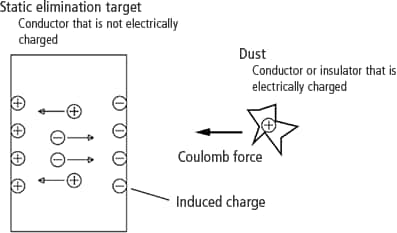Next, let us consider the case in which the object is an insulator that is not electrically charged and only the piece of dust is electrically charged. Insulators do not pass electricity. Therefore, electrostatic induction does not occur. In the Coulomb force formula given earlier, Q1 is zero, so the Coulomb force is also zero. In other words, the dust does not cling to the object.

Next [Solutions for Particle adhesion to objects]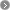•